# 4 digit by 2 digit Multiplication

### 4 digit by 2 digit Multiplication Examples

4 digit by 2 digit Multiplication – Example 1

Find the product.

2324 x 25

Explanation

Whenever we multiply a Four digit Multiplicand with two digit Multiplier, we first multiply the Multiplicand with ones place of Multiplier, and then multiply Multiplicand with Tens place of the Multiplier.

Step I : Multiply Multiplicand with ones place of Multiplier.

i.e, 2324 x 5 = 11620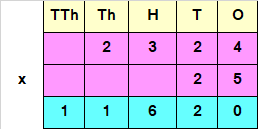Step II : Multiply Multiplicand with tens place of Multiplier.

i.e, 2324 x 2 = 4648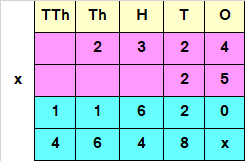Since the Number obtained at Step II, has a Tens value, we add a 0 or X at the end of the answer obtained by multiplying Multiplicand with Tens place of the Multiplier.

Step III : Add both the numbers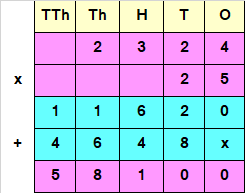Hence, 2324 x 25 = 58100

4 digit by 2 digit Multiplication – Example 2

Find the product.

9823 x 26

Explanation

Whenever we multiply a Four digit Multiplicand with two digit Multiplier, we first multiply the Multiplicand with ones place of Multiplier, and then multiply Multiplicand with Tens place of the Multiplier.

Step I : Multiply Multiplicand with ones place of Multiplier.

i.e, 9823 x 6 = 58938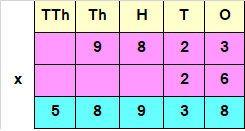Step II : Multiply Multiplicand with tens place of Multiplier.

i.e, 9823 x 2 = 19646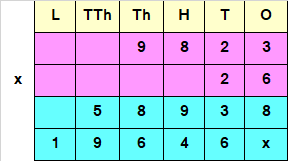Since the Number obtained at Step II, has a Tens value, we add a 0 or X at the end of the answer obtained by multiplying Multiplicand with Tens place of the Multiplier.

Step III : Add both the numbers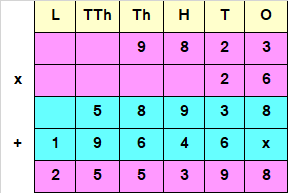Hence, 9823 x 26 = 255398